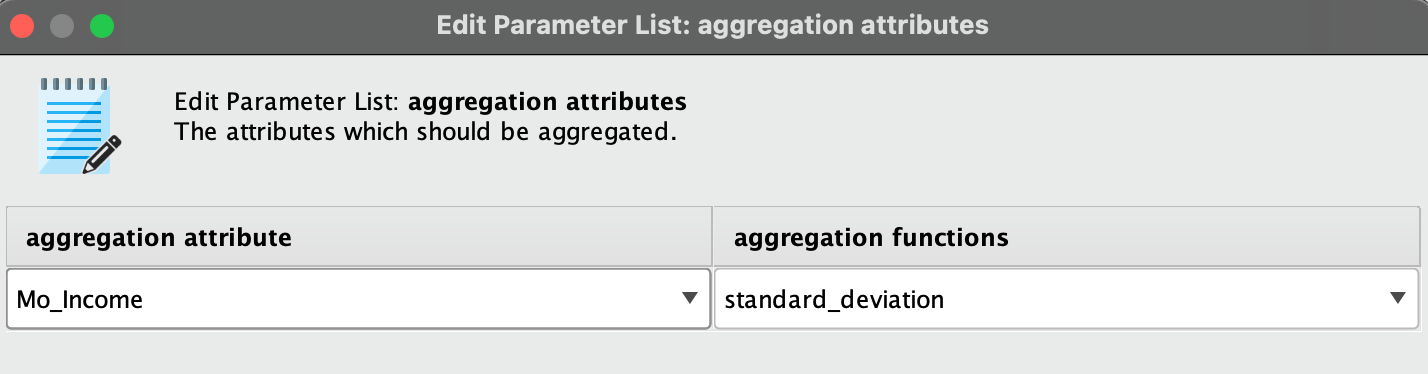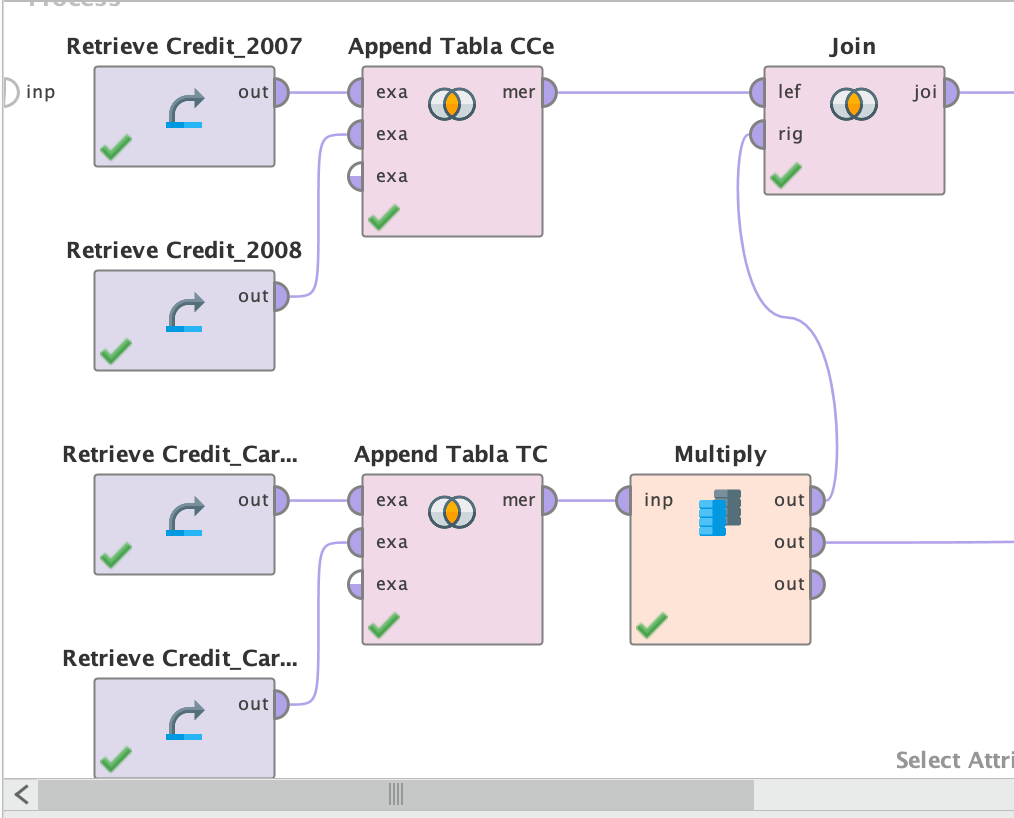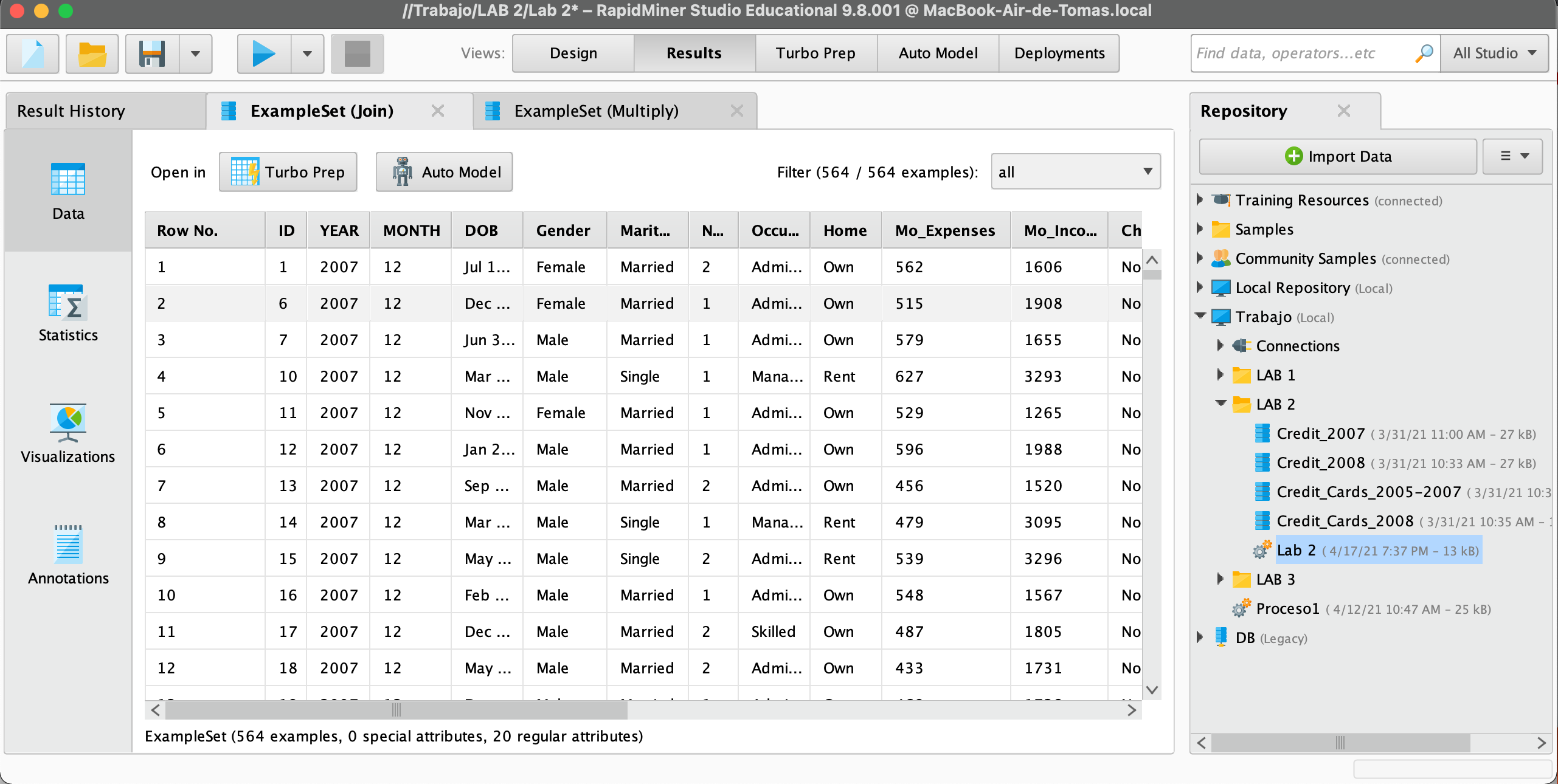# How can i create ranges for a variable?

Member Posts: 5Learner I
So, using the Aggregate function I need to make a chart presenting the standard deviation of "Mo_Income" separated by gender and by international limit. And also I will have to create ranges for the variable first.

Im doing well with the first part but i don´t know how to create the ranges for the variable

• Member Posts: 473Unicorn
Hi @Tdragi13
Can you share a sample of your data?
in order to discretize your dataset you can use, for example the Discretize by User Specification operator, (go to Cleansing->Binning).
Another option is creating  a new Attribute using logical functions and value intervals.
Best
• Member Posts: 5Learner I
Hi @ceaperez! here is a screenshot of a sample of my data!• Member Posts: 473Unicorn
Hi @Tdragi13

this is a simple example appliying the Discretize by User Specification operator.

next time, please share the process or dataset.

Best.

<?xml version="1.0" encoding="UTF-8"?><process version="9.9.000">
<context>
<input/>
<output/>
<macros/>
</context>
<operator activated="true" class="process" compatibility="9.9.000" expanded="true" name="Process">
<parameter key="logverbosity" value="init"/>
<parameter key="random_seed" value="2001"/>
<parameter key="send_mail" value="never"/>
<parameter key="process_duration_for_mail" value="30"/>
<parameter key="encoding" value="SYSTEM"/>
<process expanded="true">
<operator activated="true" class="generate_data" compatibility="9.9.000" expanded="true" height="68" name="Generate Data" width="90" x="112" y="187">
<parameter key="target_function" value="random"/>
<parameter key="number_examples" value="30"/>
<parameter key="number_of_attributes" value="1"/>
<parameter key="attributes_lower_bound" value="380.0"/>
<parameter key="attributes_upper_bound" value="650.0"/>
<parameter key="gaussian_standard_deviation" value="10.0"/>
<parameter key="use_local_random_seed" value="false"/>
<parameter key="local_random_seed" value="1992"/>
<parameter key="datamanagement" value="double_array"/>
<parameter key="data_management" value="auto"/>
</operator>
<operator activated="true" class="discretize_by_user_specification" compatibility="9.9.000" expanded="true" height="103" name="Discretize" width="90" x="380" y="187">
<parameter key="return_preprocessing_model" value="false"/>
<parameter key="create_view" value="true"/>
<parameter key="attribute_filter_type" value="single"/>
<parameter key="attribute" value="att1"/>
<parameter key="attributes" value=""/>
<parameter key="use_except_expression" value="false"/>
<parameter key="value_type" value="numeric"/>
<parameter key="use_value_type_exception" value="false"/>
<parameter key="except_value_type" value="real"/>
<parameter key="block_type" value="value_series"/>
<parameter key="use_block_type_exception" value="false"/>
<parameter key="except_block_type" value="value_series_end"/>
<parameter key="invert_selection" value="false"/>
<parameter key="include_special_attributes" value="false"/>
<list key="classes">
<parameter key="1" value="430.0"/>
<parameter key="2" value="490.0"/>
<parameter key="3" value="540.0"/>
<parameter key="4" value="650.0"/>
</list>
</operator>
<connect from_op="Generate Data" from_port="output" to_op="Discretize" to_port="example set input"/>
<connect from_op="Discretize" from_port="example set output" to_port="result 1"/>
<portSpacing port="source_input 1" spacing="0"/>
<portSpacing port="sink_result 1" spacing="0"/>
<portSpacing port="sink_result 2" spacing="0"/>
</process>
</operator>
</process>